# python学习第三课：认识python

## 目标

1. 请在 `/home/shiyanlou` 目录下创建 `shiyanlou.py` 文件
2. 在文件中输入以下内容：
``````print('hello shiyanlou')
``````
3. 进入 `Code` 目录，新建名为 `test` 的目录，然后将 `shiyanlou.py` 复制到目录 `/home/shiyanlou/Code/test` 中
4. 了解python中的print()
5. 数据类型
6. 变量
7. input()
8. 运算符

## 提示语

1. `touch` 创建文件
2. 可使用 `Sublime、gedit、Vim` 等编辑器
3. `mkdir` 创建目录
4. `cd ～` 返回 `home` 目录
5. `cp` 复制
6. 使用 `Sublime` 写入代码后，注意保存的位置。#### 知识点

• Linux 目录结构
• Linux 文件操作
• cp 命令
• mkdir 命令

python 程序的执行方式一般分为两种：

• 交互式命令行执行：在这个环境下，你输入的 Python 代码都可以立即执行，并得到输出结果，在开发中经常用于调试代码。
• 程序文件的方式执行：最常用的方式，你日常接触 99% 的程序，都是以打包成一套代码文件的方式执行的。

`>>>` 是提示符，提示你在这里输入 Python 代码。``````print('Hello World!')
``````

（ ⚠注意：️编程中标点符号要使用英文符号，请永远记住这一点 ！）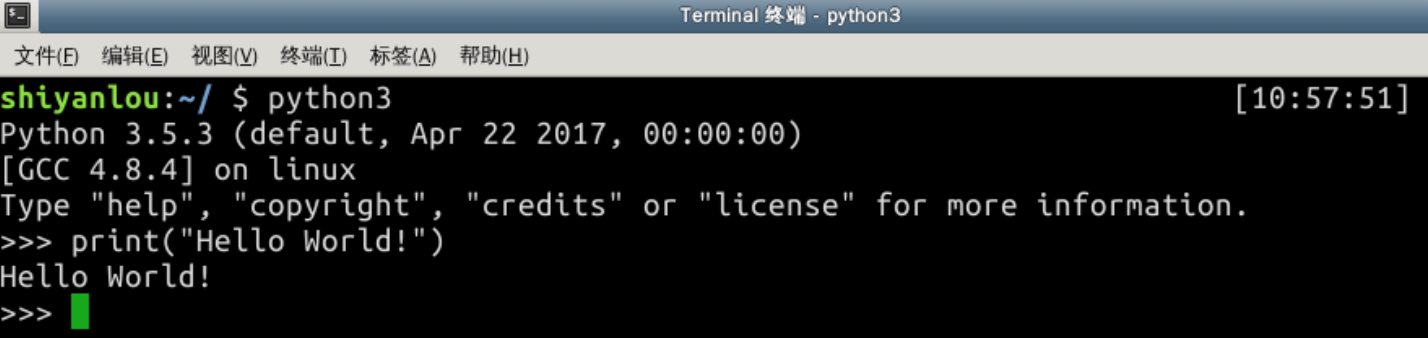print —— 打印

print('Hello World!') —— 打印 Hello World!

print() 就是你学到的第一个函数 —— 「打印函数」（函数可理解为方法），它可以帮你输出任何内容 —— 只要放进 括号 内。

``````print(1 + 1)
``````
``````print('1 + 1')
``````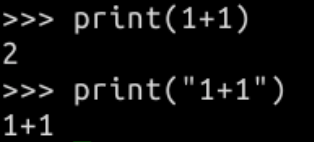⚠ ️注意：引号可以是单引号，也可以是双引号，但必须是英文符号，且前后一致。

``````print('床前明月光，疑是地上霜')
``````

``````print('''

''')
``````

``````我去买几个橘子

#### float - 浮点数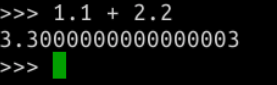#### 布尔值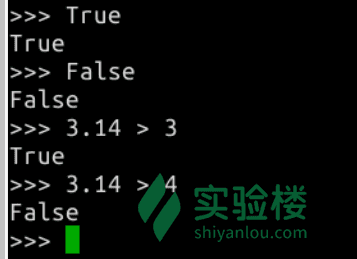#### 空值 None

`None` 在 Python 中是一个特殊的值，表示“无”。注意它与数值 0 不同，0 是一个整数，而 `无` 表示 什么都没有。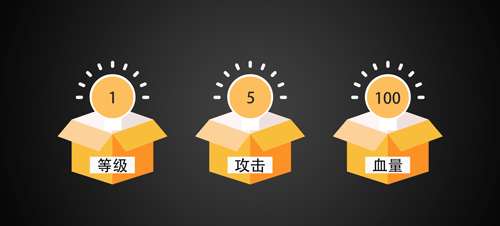``````Level = 1
ATK = 5
HP = 100
``````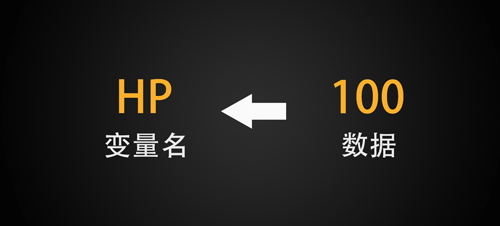x = 鸡的数量

y = 兔子的数量

x + y = 头数

2x + 4y = 脚数

``````word = 'Hello World!'
print(word)
``````

``````word2 = word
print(word2)
``````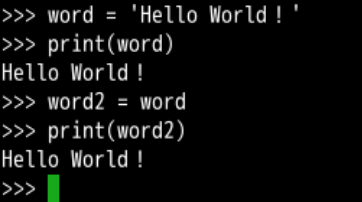“对了，还不知道你多大了，请用 Python 告诉我吧～”

``````age = input('My age is :')
``````

``````print(age)
``````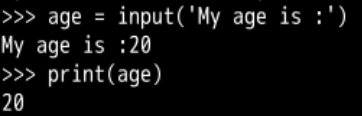``````ID = input('请输入你的ID：')
``````

（ ⚠注意️：如果input()函数执行后，你没有在后面输入内容，程序会一直卡住，等待你输入信息。）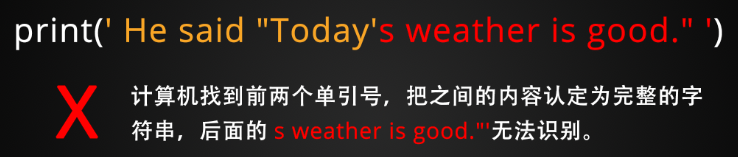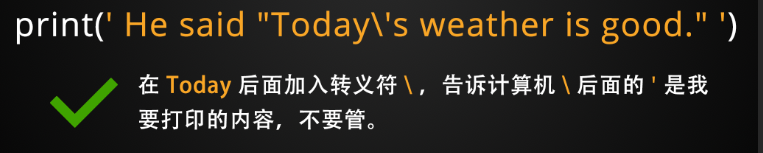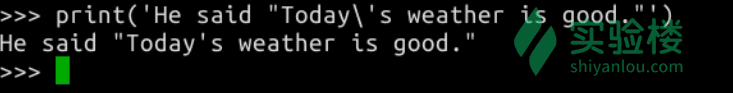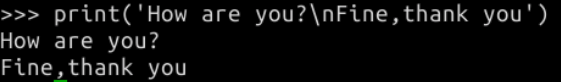#### 💡 索引

``````>>> string = 'hello_shiyanlou'
>>> string  # 获取第一个字符
'h'
>>> string  # 获取第二个字符
'e'
>>> string
'l'
>>> string[-1]  # 获取倒数第一个字符
'u'
>>> string[-2]  # 获取倒数第二个字符
'o'
``````

#### format()

`format()` 是专门用来 格式化字符串 的函数，它最常用的功能就是「插入数据」和「数字格式化」。

1. 插入数据

``````name = input('请输入姓名：')
age = input('请输入年龄')
``````

``````print('你叫'+name+'，今年' + age + '岁了')

print("我的名字叫：",name,",我今年",age,"岁了。")      # 使用逗号连接变量，输出时会出现空格

print("我的名字是"+name+",我今年"+str(age)+'岁了。')

print("我的名字叫{},今年{}岁。".format(name,age))

print("我的名字叫{a},今年{b}岁。".format(b=name,a=age))

print("我的名字叫%s,今年%d岁。"%(name,age))

print("我的名字叫%s,今年%s岁。"%(name,age)) #age强制转换成str类型了
``````

``````print('你叫{}，今年{}岁了'.format(name,age))
``````

`{}` 为占位符，我先把这个位置占住，具体数据在后面导入。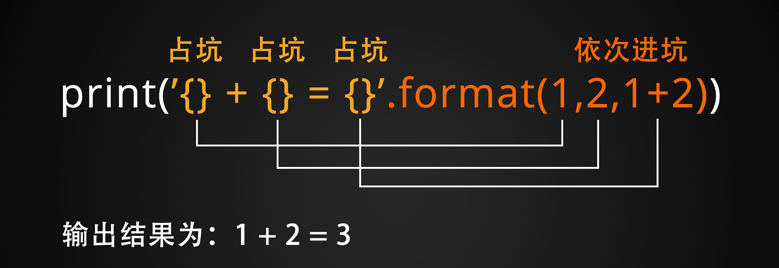2. 数字格式化

`format()` 的第二种常用功能，是格式化数字，比如我们要输出圆周率，但只保留两位小数，可以这么写：

``````print("{:.2f}".format(3.1415926))
``````

`format()`功能还有很多，我们之后用到时在做学习。

``````# 注释可以写在开头：下面要打印“hello shiyanlou”
print("hello shiyanlou")
print("hello world")  # 注释也可以写在后面：这里打印“hello world”
``````

#### 💡 算术运算符

+ 两个对象相加
- 得到负数或是一个数减去另一个数
* 两个数相乘或是返回一个被重复若干次的字符串
/ x 除以 y
% 取模 返回除法的余数
** 返回 x 的 y 次幂
// 取整除 返回商的整数部分（向下取整）

🔥 练习一下：

``````>>> a = 12
>>> b = 3
>>> c = 5
>>> a + b
15
>>> a - b
9
>>> a * b
36
>>> a / b
4.0
>>> a / c
2.4
>>> a % c
2``````

== 等于：比较对象是否相等
!= 不等于：比较两个对象是否不相等
> 大于：返回 x 是否大于 y
< 小于：返回 x 是否小于 y
>= 大于等于：返回 x 是否大于等于 y
<= 小于等于：返回 x 是否小于等于 y

⚠️ 注意： 在 Python 和大多数高级语言中：一个等号代表赋值符，两个等号才代表数学意义上的“相等”。

🔥 练习一下：

``````>>> a = 1
>>> b = 2
>>> c = 3
>>> d = 2
>>> a == b
False
>>> a != b
True
>>> a > b
False
>>> a < b
True
>>> c >= d
True
>>> b <= c
True
``````

= 赋值运算符 c = a + b ：将 a + b 的运算结果赋值为 c
+= 加法赋值运算符 c += a ：等效于 c = c + a
-= 减法赋值运算符 c -= a ：等效于 c = c - a
*= 乘法赋值运算符 c *= a ：等效于 c = c * a
/= 除法赋值运算符 c /= a ：等效于 c = c / a
%= 取模赋值运算符 c %= a ：等效于 c = c % a
**= 幂赋值运算符 c **= a ：等效于 c = c ** a
//= 取整除赋值运算符 c //= a ：等效于 c = c // a

🔥 练习一下：

``````>>> a = 3
>>> b = 12
>>> c = 10
>>> c += a
>>> print(c)
13
>>> b -= a
>>> b
9
>>> c *= 5
>>> c
65``````

and（逻辑与） a and b 当 a、b 都为 True 时，返回 True；否则返回 False
or（逻辑或） a or b 当 a、b 任意一个为 True，就返回 True
not（逻辑非） not a 当 a 为 True 时，返回 False；反之亦然

🔥 练习一下：

⚠️ 补充：在逻辑运算中，数字 `0` 代表 False，`1` 代表 True。

``````>>> not 1
False
>>> not 0
True
>>> 1 and 1
1
>>> 1 and 0
0
>>> 1 or 0
1``````

1. Python 开发环境
2. print() 函数
3. 变量与数据类型
4. input() 函数
5. 字符串
6. 运算符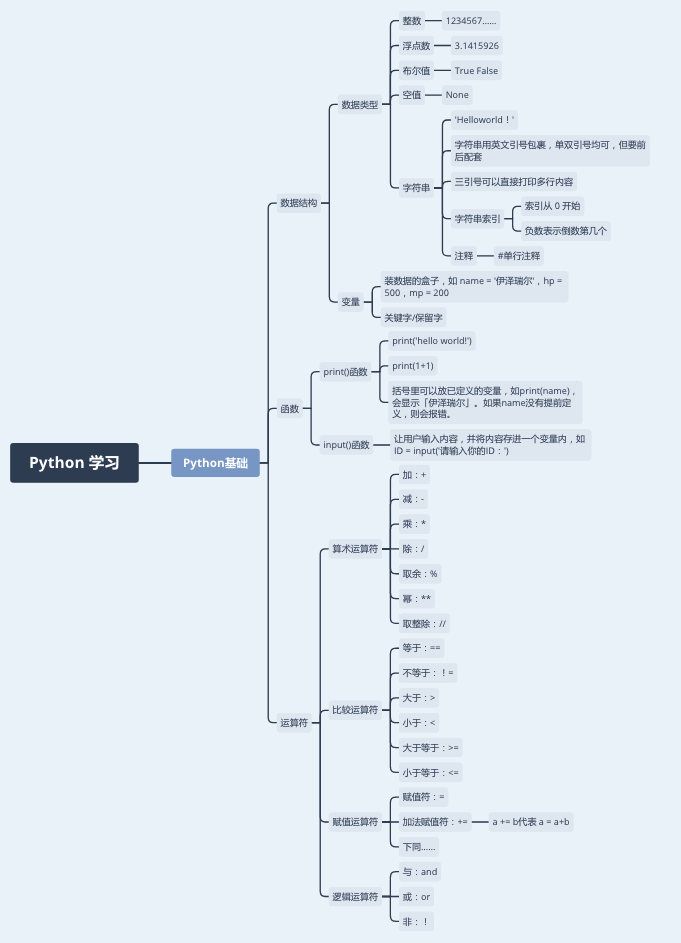Python 基础语法的三大模块中，你已经学习了「数据」「函数」两个，下节课中，下你将学习第三个模块——「流程控制」